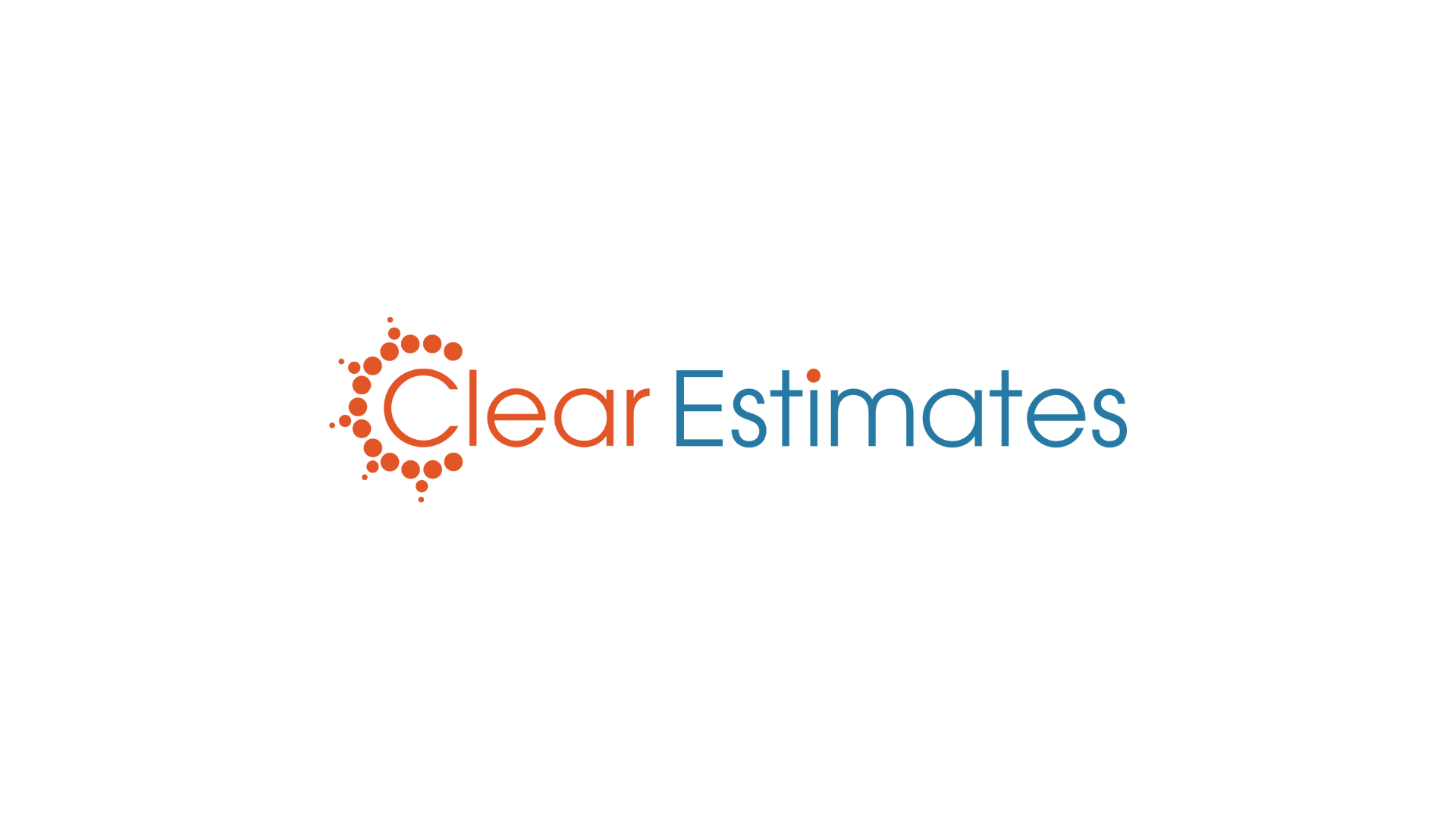Blog# Including a Discount on Your Proposal

Our Software
March 17, 2015

UPDATE 1/12/15You can now add discounts (or taxes) very easily to the proposal without using the below process. You can still use the process outlined below if you prefer, but this video will show you an easier way to do it.

We have talked before about how to add taxes to your project cost using the boilerplate, but you can use the same process for including a discount on your proposals as well. This can be useful if you want to give your customers a discount and show it very clearly on the proposal. Again, we'll be using the "Insert Fields" dropdown next to the boilerplate section where you can enter custom fields to your boilerplate.

We can use this to calculate discounts and come up with a total cost including the discount. Here are the steps:

1. Create a new boilerplate heading  in the "Outro" section called "Discount" or whatever you would like to call it.
2. Type in a short statement explaining what this section is about, such as "You have been given a discount of X% because discounts are great!"
3. Show the total project cost, the discount rate, the totaldiscount, and final "Grand Total" using the [projcost] we talked about above, An example is written up below.
4. You can multiply the project cost within the square brackets "[]".
5. For example for a 10% discount rate you can enter [projcost*.10] to calculate the amount of discount to be applied.
6. To get the total cost including taxes, just type [projcost*.90]
7. This is one minus the discount rate. So for a 5% discount you would do 1-.05 = .95. Then in your boilerplate calculate [projcost*.95] to apply a 5% discount.
8. Move this heading to the top of the "Outro" list so that it appears right below your list of line items and the project cost.
9. Thats it! Now you have an easy way to show a discount to your customers.

Here is an example of what you might type up.

#### Discount

We have applied a X% discount to your proposal based on this reason.

Project Cost: \$[projcost]

Discount: 10%

Discount in Dollars: \$[projcost*.10]

Total Project Cost: \$[projcost*.90]Jack Dean